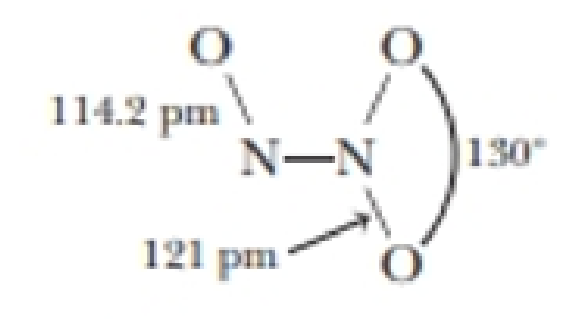Chapter 21, Problem 121SCQ

Chapter
Section
Textbook Problem

Dinitrogen trioxide, N2O3, has the structure shown here.The oxide is unstable, decomposing to NO and NO2 in the gas phase at 25 °C. N 2 O 3 (g)  →  NO(g) + NO 2 (g) (a) Draw resonance structures for N2O3 and explain why one N—O bond distance is 114.2 pm, whereas the other two bonds are longer (121 pm) and nearly equal to each other.(b) For the decomposition reaction, ΔrH° = + 40.5 kJ/mol and ΔrG° = −1.59 kl/mol. Calculate ΔS° and K for the reaction at 298 K.(c) Calculate ΔfH° forN2O3(g).

(a)

Interpretation Introduction

Interpretation: Draw resonance structures of N2O3 and it has to be explained that why one NO bond distance is 114.2pm, whereas the other two bonds are longer and nearby equal to each other.

Concept introduction:

Nitrogen forms various types of oxides on reaction with oxygen. Some of the common oxides are NO2, NO, N2O and N2O3. The oxide N2O3 is relatively unstable and decomposes to give NO and NO2. All these compounds are in gaseous state.

Resonance structures: when a single Lewis structure does not adequately represent a substance, the true structure is intermediate between two or more structures which are called resonance structures and these structures are created by moving electrons, not atoms.

Bond length is the distance between the nuclei in a bond and it is related to the sum of the covalent radii at the bonded atoms.

Bond length is inversely proportional to the bond order.

Explanation

The Lewis structure of N2O3 has three oxygen atoms attached to two nitrogen atoms.  One NO bond is a double bond and the rest two NO bonds have resonance. Thus, the resonance structures are:

(b)

Interpretation Introduction

Interpretation: The entropy change and equlibrium constant for the decomposition N2O3 has to be determined.

Concept introduction:

Nitrogen forms various types of oxides on reaction with oxygen. Some of the common oxides are NO2, NO, N2O and N2O3. The oxide N2O3 is relatively unstable and decomposes to give NO and NO2. All these compounds are in gaseous state.

Equation for Standard Gibb’s energy change is,

ΔrGorHo-TΔrSoΔrHoisthestandardenthalpychangeΔrSoisthestandardentropychange

ΔG is also related to the equilibrium constant K by the equation,

ΔrG=RTlnKp

The rearranged expression is,

Kp=eΔrGRT

(c)

Interpretation Introduction

Interpretation: The enthalpy of formation of N2O3 has to be determined.

Concept introduction:

Nitrogen forms various types of oxides on reaction with oxygen. Some of the common oxides are NO2, NO, N2O and N2O3. The oxide N2O3 is relatively unstable and decomposes to give NO and NO2. All these compounds are in gaseous state.

Equation for Standard enthalpy change ΔfHo of a reaction can be calculted using the equation,

ΔrH°=nΔfH°(products)nΔfH°(reactants)

Still sussing out bartleby?

Check out a sample textbook solution.

See a sample solution

The Solution to Your Study Problems

Bartleby provides explanations to thousands of textbook problems written by our experts, many with advanced degrees!

Get Started

Name the following compounds. a Sn3(PO4)2 b NH4NO2 c Mg(OH)2 d NiSO3

General Chemistry - Standalone book (MindTap Course List)

Water-soluble vitamins are mostly absorbed into the lymph. the blood. the extracellular fluid, b and c.

Nutrition: Concepts and Controversies - Standalone book (MindTap Course List)

What is physically exchanged during crossing over?

Human Heredity: Principles and Issues (MindTap Course List)